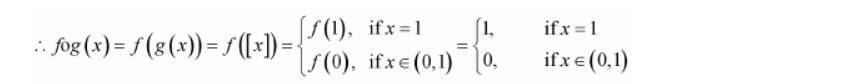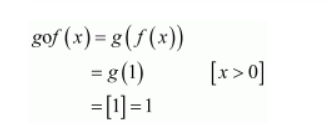# Let f: R → R be the Signum Function defined as

Question:

Let $f: \mathbf{R} \rightarrow \mathbf{R}$ be the Signum Function defined as

f(x)=\left\{\begin{aligned} 1, & x>0 \\ 0, & x=0 \\-1, & x<0 \end{aligned}\right.

and $g: \mathbf{R} \rightarrow \mathbf{R}$ be the Greatest Integer Function given by $g(x)=[x]$, where $[x]$ is greatest integer less than or equal to $x$. Then does fog and gof coincide in $(0,1]$ ?

Solution:

It is given that,

$f: \mathbf{R} \rightarrow \mathbf{R}$ is defined as $f(x)=\left\{\begin{array}{rr}1, & x>0 \\ 0, & x=0 \\ -1, & x<0\end{array}\right.$

Also, $g: \mathbf{R} \rightarrow \mathbf{R}$ is defined as $g(x)=[x]$, where $[x]$ is the greatest integer less than or equal to $x$.

Now, let $x \in(0,1]$.

Then, we have:

$[x]=1$ if $x=1$ and $[x]=0$ if $0Thus, when$x \in(0,1)$, we have$f \circ g(x)=0$and$g \circ f(x)=1$. Hence, fog and gof do not coincide in$(0,1]\$.